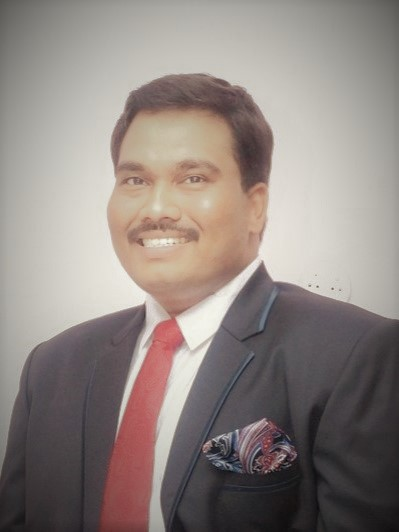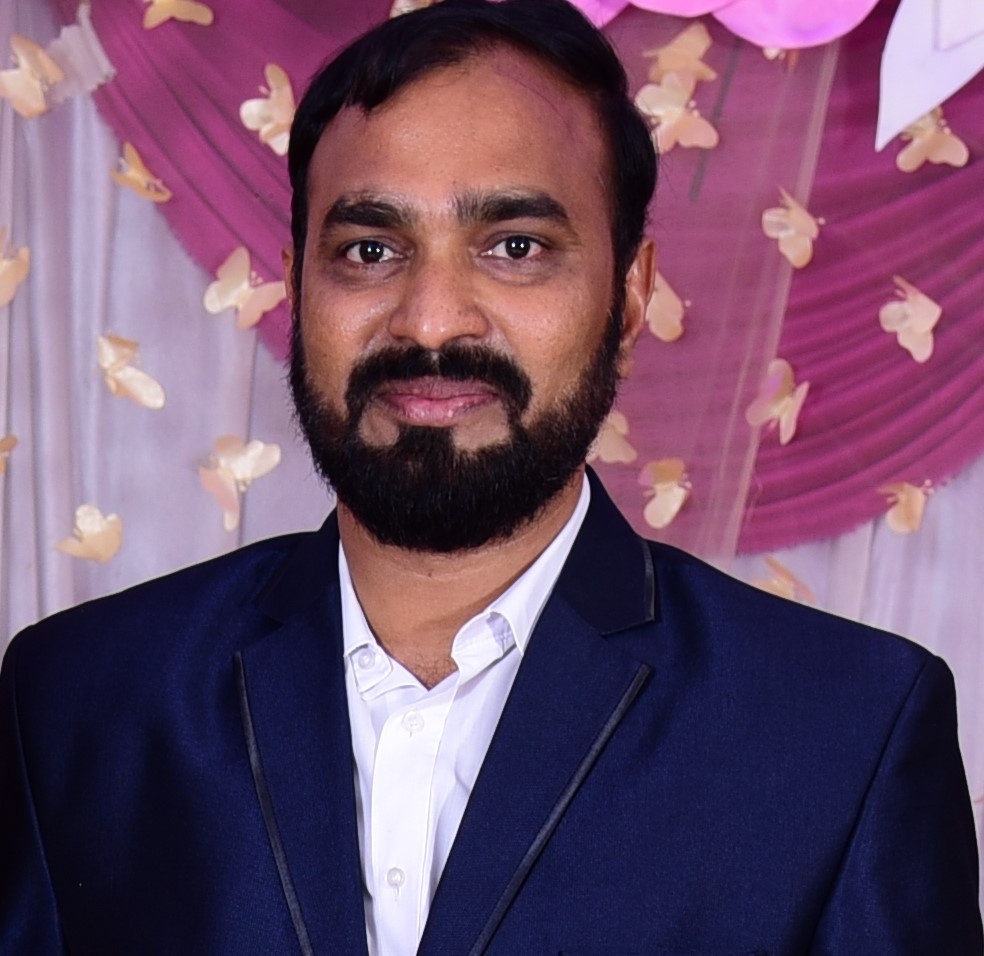# Electromagnetic Field Theory and Transmission LinesTeacher

##### Dr P Anthony Sunny DayalCategory

### Course Attendees

Still no participant

Still no reviews

# Code(Credit) : CUTM1042 (2-1-0)

## Course Objectives

• To introduce the fundamental theory and concepts of electromagnetic waves and transmission lines
• To impart knowledge on the concepts of electrostatics, electric potential, energy density and their applications.
• To impart knowledge on the concepts of magnetostatics, magnetic flux density, scalar and vector potential and its applications.
• To impart knowledge on the concepts of Faraday‘s law, induced emf and Maxwell‘s equations.
• Model and design the transmission lines at high frequencies.
• To apply Smith chart use for solution of transmission line problems and impedance matching.

## Learning Outcomes

• Apply the principles of electrostatics to the solutions of problems relating to electric field and electric potential.
• Apply the principles of electrostatics to the solutions of problems relating to boundary conditions and electric energy density.
• Apply the principles of magnetostatics to the solutions of problems relating to magnetic field and magnetic potential,
• Apply the principles of magnetostatics to the solutions of problems relating to boundary conditions and magnetic energy density.
• Understand the concepts related to Faraday‘s law, induced emf and Maxwell‘s equations.
• Apply Maxwell‘s equations to solutions of problems relating to transmission lines and uniform plane wave propagation.

## Course Syllabus

Module I: Electrostatics (3hrs Theory + 2hrs Practice)
Introduction to Electrostatic Fields, Gauss's Law and Applications, Electric Potential, Maxwell’s Two Equations for Electrostatic Fields,Electric Current and Current Density, Continuity Equation, Relaxation Time, Laplace’s and Poisson’s Equations.

Practice:
1. To Calculate the Electric field of a dipole using Coulomb's law in Matlab
2. Simulation of Electric Potential and Electric Field in Matlab

Module II: Magnetostatics (3hrs Theory + 2hrs Practice)
Biot-Savart Law: Current Flow – which path does it take, Ampere’s Circuital Law, Magnetic Flux Density: Closed Loop Circuits, Magnetic Scalar and Vector Potentials, Forces due to Magnetic Fields, Inductances and Magnetic Energy.

Practice:
1. Magnetic field by an infinitely long line current using matlab
2. Magnetic field of a Circular current loop using Biot Savart's Law

Module III: Maxwell’s Equations (3hrs Theory + 1hr Practice)
Maxwell’s Equations and Boundary Conditions.

Practice:
1. Maxwell's Equation using matlab

Module IV: Electromagnetic Waves (3hrs Theory + 4hrs Practice)
Wave Equations for Conducting and Perfect Dielectric Media, Uniform Plane Waves, Wave Propagation in Lossless and Conducting Media, Polarization, Reflection and Refraction of Plane Waves – Normal and Oblique Incidences for both Perfect Conductor and Perfect Dielectrics, Brewster Angle, Critical Angle and Total Internal Reflection, Surface Impedance. Poynting Vector and Poynting Theorem.

Practice:
1. Linear and Circular Polarization of waves using matlab
2. 1-D standing wave using matlab
3. 2-D standing wave (TE) using matlab
4. 2-D standing wave (TM) using matlab
5. Design of Wireless Power Transfer using matlab

Module V: Introduction to Transmission Line Modelling (3hrs Theory + 3hrs Practice)
Introduction to Transmission line equations, Primary & Secondary constants Expressions for Characteristic Impedance, Propagation Constant, Phase and Group Velocities, Losslessness/Low Loss Characterization, Distortion , Loading, Transmission Line Effects, SC and OC Lines, Reflection Coefficient, VSWR, λ/8, λ/4, λ/2 line impedance Transformations, Smith Chart – Configuration and Applications, Impedance Control.

Practice:
1. Reflection and transmission of a plane wave (S-wave)
2. Reflection and transmission of a plane wave (P-wave)
3. Radiation by an infinitesimal dipole

Module VI: Waveguides (3hrs Theory)
Introduction, Rectangular Waveguides, electric and magnetic field patterns in TE10 and TE11 mode configuration, modes of TE wave in rectangular waveguide, field equations, impossibility of TEM wave propagation in waveguides, cutoff frequency of rectangular waveguide, propagation constant, wave impedance, phase velocity, group velocity, dominant mode and degenerate modes, related problems.

Module VII: Electromagnetic Computational Techniques (3hrs Theory)
Introduction, Finite Difference Method (FDM), Finite Element Method (FEM) and Method of moments (MOM) technique.

Text Books:
1. Matthew N.O. Sadiku, “Elements of Electromagnetics”, Oxford Univ. Press.
2. G.S.N.Raju, “Electromagnetic Field Theory and Transmission Lines”, Pearson Education (Singapore) Pvt., Ltd.

Reference:
1. E.C. Jordan and K.G. Balmain, “Electromagnetic Waves and Radiating Systems”, PHI.
2. Seungbum Hong, "Electrodynamics: An Introduction", Coursera.
3. Seungbum Hong, "Electrodynamics: Electric and Magnetic Field", Coursera.
4. Seungbum Hong, "Electrodynamics: In-depth Solutions for Maxwell’s Equations", Coursera.
5. Husain Habib, "Electromagnetic Tutorials part 1 with MATLAB & GeoGebra", Udemy.

## Session 3

Continuity Equation and Relaxation Time

Laplace’s and Poisson’s Equations

## Session 6

Magnetic Scalar and Vector Potentials

## Session 7

Ampere’s Circuit Law, Magnetic Flux Density, Magnetic Scalar and Vector Potentials

## Session 8

Forces due to Magnetic Fields, Inductance and Magnetic Energy

## Session 10

### Our Main Teachers### Dr P Anthony Sunny Dayal

##### Associate Professor, Department of Electronics and Communication Engineering, SOET
VIEW PROFILE

Dr P Anthony Sunny Dayal completed his M.Tech in Radar and Microwave Engineering from Andhra University and Ph.D in Antenna Array Design For Radar And Emc Applications from Centurion University. Recipient of “Young Scientist Award” from His Excellency Shri Biswabhusan Harichandan Governor of Andhra Pradesh, at Andhra Pradesh Science Congress – 2019 by Andhra Pradesh […]### Dr Murali Malijeddi

##### Professor
VIEW PROFILE

Dr Murali M, Working as a Professor in the Department of ECE, School of Engineering , Centurion University , Andhra Pradesh. He did his PhD from Andhra University , M.E in Control Systems, Andhra University College of Engineering, B.Tech in Electronics and Communication Engg, Siddartha Engineering College, Vijayawada. He had 20 years of teaching and research experience and discharged his duties at various manager levels such as Head of the department and also as Principal for an Engineering college.He organized and participated in several national International Technical conferences,He presented and published 20 International and 8 National research papers, presently guiding 4 PhD scholars and his research area of interest includes advanced antennas design for radar and satellite communication , wireless communications and networks.

### Abinash Rath

##### Assistant Professor
VIEW PROFILE

I have received my B. Tech in Electrical Engineering from Government College of Engineering Keonjhar, India and M. Tech in Power Electronics Control and Drives from VSSUT, Burla, India. I am pursuing my Ph.D. in the Department of Electrical Engineering, National Institute of Technology Rourkela, India. His fields of interest include power electronic converters, shunt […]